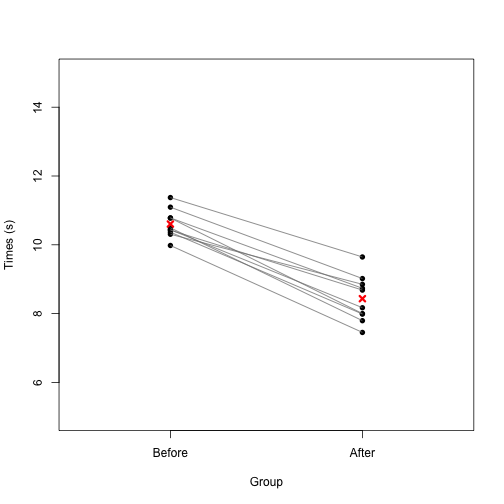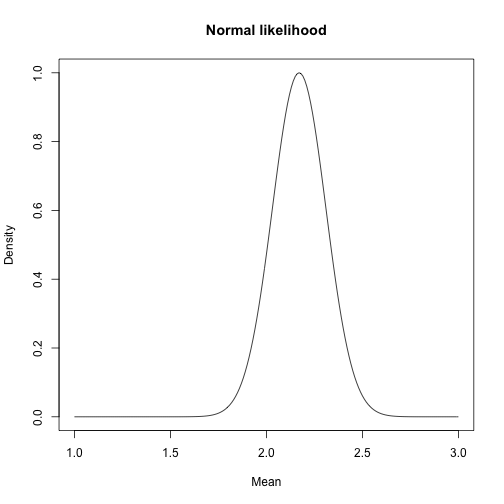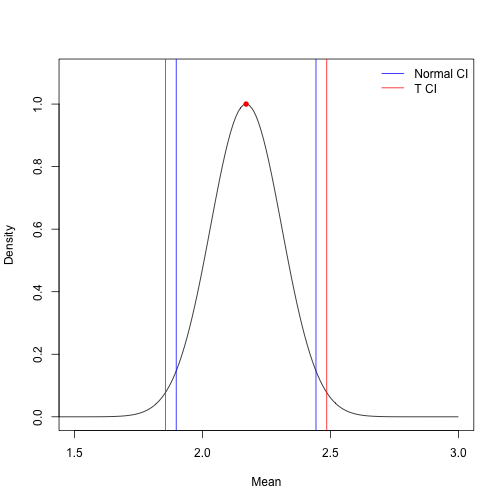# Exercise 2: Normal distribution/T-Tests

## Instructions:

The same as Exercise 1.

Hints and reminders are italic

Questions appear in blue.

Exercise to be handed in by 7th February

## Question vocabulary:

• Describe is asking you to summarise some outputs or findings e.g. “X is larger than Y” or “X has a straight line relationship with Y with some outliers” or “The confidence interval is 2.4 to 3.1”
• Interpret is asking you to take what you can describe and put it into context (especially back into the units it was calculated from) e.g. “X is larger than Y by 5 days, this means that…” or “X has a straight line relationship with Y of 1.5 degrees to every day change in X. This shows that it will be 10.5 degrees warmer at the end of the week” or “The confidence interval is 2.4 to 3.1, meaning we are 95% confident that the population mean lies between these values. The CI does not span zero so we are confident our estimate is statistcally different to zero.”
• Your opinion if we ask what you think then we need an opinion as an answer. Give reasons to your answer, there may not be a clear wrong or right. But you should use data available to back up your opinion.

## Resources:

• Chapter 3 in 'The New Statistics with R'
• Google (or any other search engine)

## The challenge: Should you try to outrun a zombie?

It is day 40 after the event. An infection has been spreading through the world. Its symptoms produce a zombie-like state of reduced cognitive function coupled with increased agression. 50% of the population have already been infected. It is not known how many are immune.

You are a group of statisticians who have managed to remain safe inside your office. You now need to decide whether to stay here or try and run to a different location. But, can you outrun the zombies? From TV and film you have pieced together mixed information on zombie abilities. Some sources suggest zombies are slow, only walking or shuffling. Others show that zombies can move incredibly fast, even running. The only way we can find out how these zombies behave is with data.

Luckily! One of your team has a dataset that might help.

One infection outbreak began at the 2018 athletics tournament in Paris. The 100m final had been run two days before when an outbreak occurred during the 100m relay. For some reason the newly infected athletes still decided to run the relay event (we don't know why). So you have data on the 100m times for the same runners both before and after infection.The data can be found here: https://www.math.ntnu.no/emner/ST2304/2019v/Week4/Ztimes100.csv

It is your job to analyse the data and decide whether to run or stay.

The first step is to import the data and assign it to an object. You can use the whole web link above to import the data. It is a csv file with column names (header) included.

The next step is to plot the data, we always want to look at our data before we start doing anything else.

We have made a graph for you (you do not need to know all the code used to do it). The grey lines show which times are from the same athlete. The means are marked in red.To analyse this data you will be using a paired t-test.

1. Why does the t-test need to be paired?

2. What are you trying to find out using a t-test? ( What does it model?)

T-test can be conducted in several ways in R:

The first way is to use lm() function. Take a look at the function using ?lm. The arguments you will use in lm are x, and y. lm(y ~ x)

You will need to create a vector of differences between the times before and after infection. This will make the test paired. This will be y.

x here is 1 as we are looking only at the mean. It makes it an intercept only model.

Remember: which column you substract from the other will influence how you interpret the answer

``````# Create a vector of paired differences
# the - symbol automatically subtracts each element of a vector from the
# same location in another vector (creates paired differences)
differences <- Ztimes100\$times_before - Ztimes100\$times_after

# run a paired t-test on the differences between before and after 100m times.
t_test1 <- lm(differences ~ 1)

# show the output of the lm, this displays:
# - model you have run
# - the quantiles of the residuals (distance between data and estimate)
# - values of the coefficient (here:
# * estimate = the mean difference
# * Std. Error = the standard error of the mean
# * t value = the calculated t statistic
# * Pr(>|t|) = probability value)
# - Significance codes (ignore these)
# - Residual error and degrees of freedom
summary(t_test1)
``````
``````##
## Call:
## lm(formula = differences ~ 1)
##
## Residuals:
##     Min      1Q  Median      3Q     Max
## -0.7138 -0.3520  0.0018  0.3205  0.6097
##
## Coefficients:
##             Estimate Std. Error t value Pr(>|t|)
## (Intercept)   2.1707     0.1393   15.58  8.1e-08 ***
## ---
## Signif. codes:  0 '***' 0.001 '**' 0.01 '*' 0.05 '.' 0.1 ' ' 1
##
## Residual standard error: 0.4405 on 9 degrees of freedom
``````
``````# We can show the rounded 95% confidence intervals like this
round(confint(t_test1),2)
``````
``````##             2.5 % 97.5 %
## (Intercept)  1.86   2.49
``````

3. What are the confidence intervals for this t-test (what values are the upper and lower bound)? Describe what they mean.

The second way is by using R as a calculator.

The formula for a t-test is:

mean of data/the standard error

The formula for the standard error is:

the standard deviation/the square root of sample size (n)

4. Using the formulas above, calculate the T statistic in R. What value do you get? (include a copy and paste of your code)

Have a look at how your results from question 4 and compare to the results from lm(). Hopefully they will be the same. This is one way to check you did question 4 correctly.

5. Your team must decide whether to stay or go. Based on the data you have analysed, do you decide to try and outrun the zombies? Include reasons and the results in your answer (mean and CIs). hint: think about what the mean difference you calculated means in terms of 100m times

6. Do you think you could you have used a normal distribution here instead of a t-distribution? Say why/why not.

One way to test whether using a normal distribution instead of a T distribution would work is to try it!

7. How many parameters does the normal distribution have?

To model the differences in 100m times as a normal distribution you can use the dnorm() function (similar to what we did last week with the Binomial and Poisson distributions). This allows us to calculate the likelihood, which for a continuous distribution is the density.

``````# First we write a short function that lets us sum the
# log likelihoods for all data points to give one
# likelihood measure for the whole dataset

# this line sets up the function
Likelihood_calculation <- function(data, mean, sd) {
# this line sums the log likelihoods
likelihood <- sum(dnorm(data, mean, sd, log=TRUE))
# this line returns the likehood for the whole dataset
return(likelihood)
}

# next set up a vector of different mean values to
# get the likelihood for
means <- seq(1,3,0.01)

# Now use the 'Likelihood_calculation' function we made earlier.
# Use it inside a function called sapply.
# You don't need to know much about this function,
# but it runs another function for all values of a vector (here 'means')
likelihoods.norm <- sapply(means, Likelihood_calculation,
data = differences, sd = sd(differences))

# We can look at a few of the likelihoods (exp back transforms the log)
``````
``````##  1.899876e-18 3.464348e-18 6.284629e-18 1.134226e-17 2.036486e-17
##  3.637684e-17
``````
``````# WOW! Those are really small numbers.
# So when we plot we subtract the maximum likelihood to scale the
# numbers and make them a bit more relatable.
# plot the outcome
plot(means, exp(likelihoods.norm-max(likelihoods.norm)),
type="l", xlab="Mean", ylab="Density", main = "Normal likelihood")
````````````# Optimise the maximum likelihood for the norm distribution
# this code works the same way as optimise did in Exercise 1
MLE_norm <- optimise(Likelihood_calculation, lower = 0, upper = 10,
data = differences,
sd = sd(differences), maximum = TRUE)\$maximum

# show the rounded result
round(MLE_norm,2)
``````
``````##  2.17
``````

You have found that the maximum likelihood estimate for the mean of the differences is 2.17. It is not surprising that this is the same as the mean difference as calculated by the t-tests above. But is the estimate of uncertainty the same? To find out we need to calculate the confidence intervals.

``````# Calculate the confidence intervals for our normal maximum likelihood
# estimate.

# First save an object that is the standard error
standard_error_norm <- sd(differences)/sqrt(length(differences))

# Then ± 1.96 times the standard error from the
# maximum likelihood estimate
CI_upper <- MLE_norm + 1.96*standard_error_norm
CI_lower <- MLE_norm - 1.96*standard_error_norm
# display the confidence intervals to 2 decimal places
round(c(CI_lower, CI_upper),2)
``````
``````##  1.90 2.44
``````

The confidence interval calculated from the Normal distribution is 1.89 to 2.45.

8. Compare these confidence intervals those calculated from the T distribution?

You should have those written down from question 3. But we will print them below too.

``````# To extract only the confidence intervals from the lm() output
# we can use the code below.
round(confint(t_test1),2)
``````
``````##             2.5 % 97.5 %
## (Intercept)  1.86   2.49
``````

You can also plot the difference confidence intervals. Here we plot them both on the Normal distribution density plot.

``````# First create a plot with the Normal distribution density.
# We use xlim and ylim to set the limits of our axes to make
# the plot look nicer and be easier to read.
plot(means, exp(likelihoods.norm-max(likelihoods.norm)),
type="l", ylab="Density", xlab="Mean", xlim = c(1.5,3), ylim=c(0,1.1))
# We then add a point for the maximum likelihood estimate of the mean.
points(MLE_norm, 1, col = "red", pch=16)

# Then add the T distribution confidence intervals in red
abline(v = c(confint(t_test1), confint(t_test1)), col = "red")
# and the Normal distribution confidence intervals in blue
abline(v = c(CI_lower, CI_upper), col = "blue")

# The final step is a legend to explain the colour scheme
# bty = 'n' just means there will be no box around the legend
legend("topright", c("Normal CI", "T CI"), bty = "n",
lty= c(1,1), col = c("blue", "red"))
``````9. Now you have tried the Normal distribution, do you think the Normal distribution is a good model for this data? Why/Why not In what situation might it become better?

10. Consider the Normal distribution confidence intervals. If you use these only, would it change your decision to run or stay (question 5)? Explain your answer

11. What other data might you want to improve your decision making? Could there be any problems with the current study design?